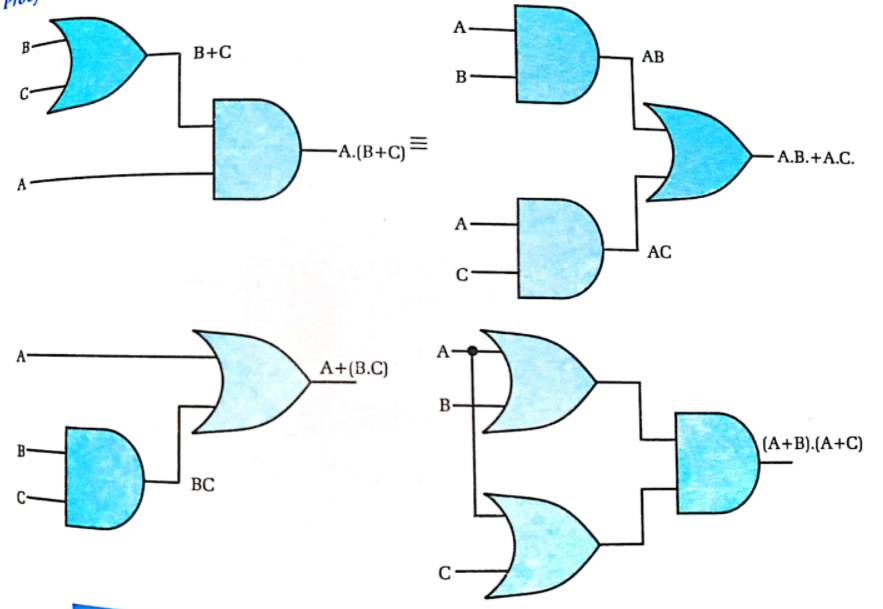Distributive LawIt states that the multiplication of two variables and adding the result with a variable will result in the same value as multiplication of addition of the variable with individual variables. Likewise, it also states that the sum of two variables and multiplying the result with a variable will result in the same value as sum of multiplication of the variable with individual variables.
i.e.,
➡ A.(B + C) = A.B + A.C
➡ A + (B.C) = (A + B) . (A + C)
Proof:Hence, A.(B + C) = A.B + A.C & A + (B.C) = (A + B) . (A + C)